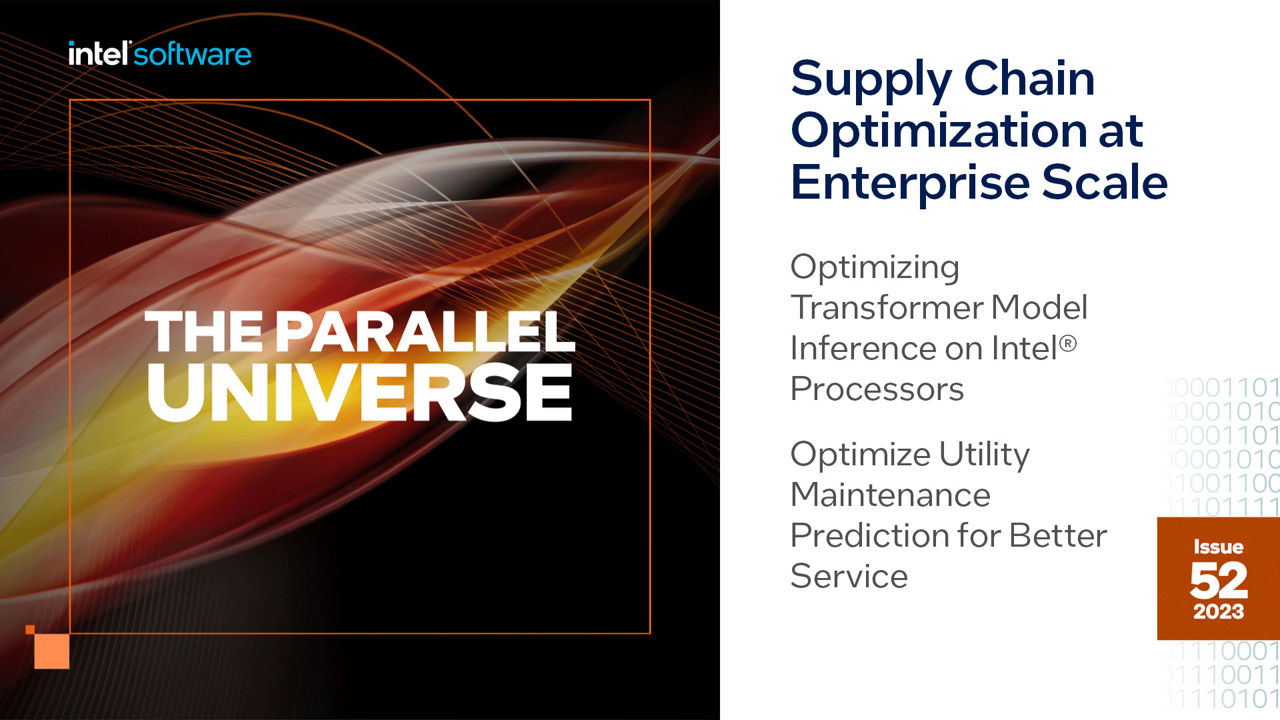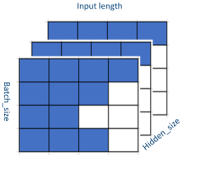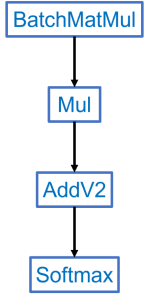# Optimizing Transformer Model Inference on Intel® Processors

Fusing Operations to Take Advantage of the oneAPI Deep Neural Network (oneDNN) Library

## Get the Latest on All Things CODEBy

The Transformer model is one of the most popular models in natural language processing (NLP) (Figure 1). Since its publication by Google in 2017, it has been adopted by many other NLP models, and even extended to non-NLP models. Language translation is a common use of Transformer, largely replacing the LSTM models that were used previously, because of its better accuracy and parallelism. The BERT model is based on Transformer, so optimizing Transformer can improve many NLP and non-NLP models.Figure 1. The Transformer model architecture (Source: Vaswani et al., Attention Is All You Need)

Starting with the Transformer training and evaluation code from MLCommons (v0.5), Intel has optimized the model for inference on Intel® Xeon® Scalable processors. These optimizations achieve significant performance improvement for both inference throughput and latency. The optimized model has been added to the Model Zoo for Intel® Architecture.

## Training Models

Before attempting to optimize for inference, we must first get a trained model. We started with the MLPerf* Transformer model (v0.5), trained to the accuracy suggested by MLPerf. We obtained checkpoints for the model, which can be further trained for slightly better accuracy, if needed. Although inference can be run from the trained checkpoints, it is usually a suboptimal way to deploy the model because the size of the checkpoints is large because they contain training nodes, which are not needed for inference. Running inference from checkpoints also adds overhead from weight loading.

To optimize inference performance, instead of using the trained checkpoints, we freeze the trained model checkpoints into a graph that only contains the inference graph and the model weights. As a result, the MLPerf Transformer model size is reduced to around 800 MB, compared to more than 1 GB with checkpoints. More importantly, the frozen graph is necessary if users want to quantize the model to int8 for an additional performance boost. The script to freeze the graph is available in the Model Zoo for Intel Architecture.

In the process of freezing the graph, the graph has gone through a few optimizations, like constants folding, identity node removal, and so on. After freezing the graph, we obtained a protobuf (.pb) file with known input and output nodes. It is quite simple to run the inference from the frozen graph. Users just need to feed input data into the input nodes of the model. After execution, the results are obtained from output nodes of the model. More importantly, if users have ideas to further optimize the model later, they can refreeze the model with the optimized model code without retraining, as long as the weights of the model are unchanged.

The Transformer model has two major modules: an encoder and a decoder. Each module has multiple layers: feed forward network (FFN), encoder-decoder attention, beam search, ops fusion, embedding, self-attention, etc. We will demonstrate the optimizations that we’ve done for several of these layers.

The most important optimization that we’ve done is to remove the padding/unpadding algorithm from the FFN layer. The input to this layer is a 3D padded tensor with shape [batch_size, input_length, Hidden_size]. The model has paddings in the second dimension, as shown in Figure 2, where blank blocks denote the padding value, which is zero. The model finds and removes the paddings before feeding the tensor into the dense layer and reshapes the tensor to 2D with shape [batch_size, number_tokens*Hidden_size], as shown in Figure 3. There is no reason to compute the values at the paddings because they will still be zero. However, after the dense layer, the model needs to put the padding back into the output tensor and restore the original shape (because it will be the input tensor for the next layer).Figure 2. Data layout of the FFN input tensorFigure 3. Optimized data layout of the FFN input tensor

The padding/unpadding algorithm tries to reduce the amount of computation by using more memory operations. This can improve performance if the computations are slower than memory operations. However, in modern Intel Xeon Scalable processors, computation is faster than the memory operations for this algorithm. Therefore, removing padding/unpadding from the FFN layer improves performance.

## Encoder-Decoder Attention Cache Optimization

In the Transformer model, when the input tensor goes through the encoder module, the output is an encoder memory tensor, which will be used in the decoder module to compute encoder-decoder attention (Figure 4).Figure 4. Encoder-decoder attention

Attention is the most important part of the Transformer model. It is computed with the following formula, where Q, K, and V are the tensors after dense layers:

$$Attention(Q,K,V)=softmax(\frac{QK^T}{√{d_k}})V$$

Q is the result of the dense layer with the decoder’s input. K and V are the results of the dense layers with the input of the encoder memory tensor. In every step of the decoder iterations, the value of the input tensor of the decoder changes, but the values of the memory tensor from the encoder stay the same. That means the K and V tensors stay the same in every step of the decoder, but the original Transformer model recomputes these tensors.

Obviously, this redundant computation should be eliminated. To remove it, we added a mechanism to cache K and V. We only compute the K and V tensors at the beginning of encoder-decoder attention, then cache the values to reuse in the remaining decoder iterations.

## Beam Search Cache Optimization

Beam search is another time-consuming Transformer module. Profiling shows that the biggest bottleneck in this module is the Gather_ND operation, which collects tensor values that satisfy certain conditions (Figure 5). The random-access memory pattern makes this operation hard to vectorize and parallelize.Figure 5. Gather_ND pattern

However, we noticed that the values we need to collect in the model are vectors in a multidimension tensor. The TensorFlow* framework includes a Gather_V2 operation that collects vectors from a tensor (Figure 6). This operation is easier to vectorize and parallelize, but the results should be the same. Replacing Gather_ND with Gather_V2 in the beam search algorithm improves performance.Figure 6. Gather_V2 pattern

## Ops Fusion Optimization

Ops fusion is a common optimization in deep learning models. In Transformer, we noticed that MatMul, Reshape, BiasAdd, and element-wise operations are hotspots in the FFN layer. Intel optimizations in TensorFlow fuse certain patterns of these operations into a new operation. However, the pattern of operations in the Transformer model (Figure 7) don’t match the required fusion pattern (Figure 8). After investigating the model source code, we found that we can move the Reshape operation before MatMul without causing any errors or increasing computation or memory usage. This can be done by changing just a few lines in the source code.Figure 7. Operations before optimizationFigure 8. Modified pattern

When we run inference with the regenerated model, the Intel® Optimization for TensorFlow* runtime automatically fuses operations to a new operation named MklFusedMatMul (Figure 9), which calls the highly optimized oneAPI Deep Neural Network Library (oneDNN). This reduces memory operations and achieves much better performance than the original model.Figure 9. Final fused optimized pattern

We noticed that the performance hotspots for inference in the Transformer attention layer are Mul, BatchMatMul, AddV2, and Softmax (Figure 10). Mul, AddV2, and Softmax are element-wise, memory-bound operations.Figure 10. Original sequence of operations

The Intel optimizations in TensorFlow fuse the BatchMatMul, Mul, and AddV2 sequential operations. Once again, rearranging the pattern (as shown in Figure 11) exposes an optimization opportunity (Figure 12). These fusions are important to the model—not only to compute more efficiently but also reduce memory traffic, which is especially important for memory-bound operations.Figure 11. Modified sequence of operationsFigure 12. Final fused optimized pattern

The next optimization is to fuse layer normalization in the Transformer model. Layer normalization is used in every layer of the encoder and decoder modules. These operations are either memory movement or element-wise operations. They are memory-bound, performance bottlenecks, so to reduce memory traffic for layer normalization, we implemented the layer normalization fusion (Figures 13 and 14).Figure 13. Operations before optimization (left) and Figure 14. Optimized pattern (right)

## Concluding Remarks

Transformer is a powerful but complicated deep learning model. The Transformer inference model in the MLPerf repository does not perform as well as the Intel-optimized version that takes advantage of the oneDNN library. The optimizations described above are based on the default FP32 model, which gave us the baseline inference performance. They significantly improved performance without sacrificing model accuracy. The model can be further optimized by converting to bfloat16 and int8 on supporting hardware. For example, the 4th Generation Intel Xeon processor supports both bfloat16 (BF16) and 8-bit quantization (int8) using Advanced Matrix Extension (AMX) instructions:

With converted models using these reduced precision supports, we can achieve even better performance. For more information, please refer to the Model Zoo for Intel Architecture. We have published all trained checkpoints, frozen graphs, and source code in the repository for your reference and use.